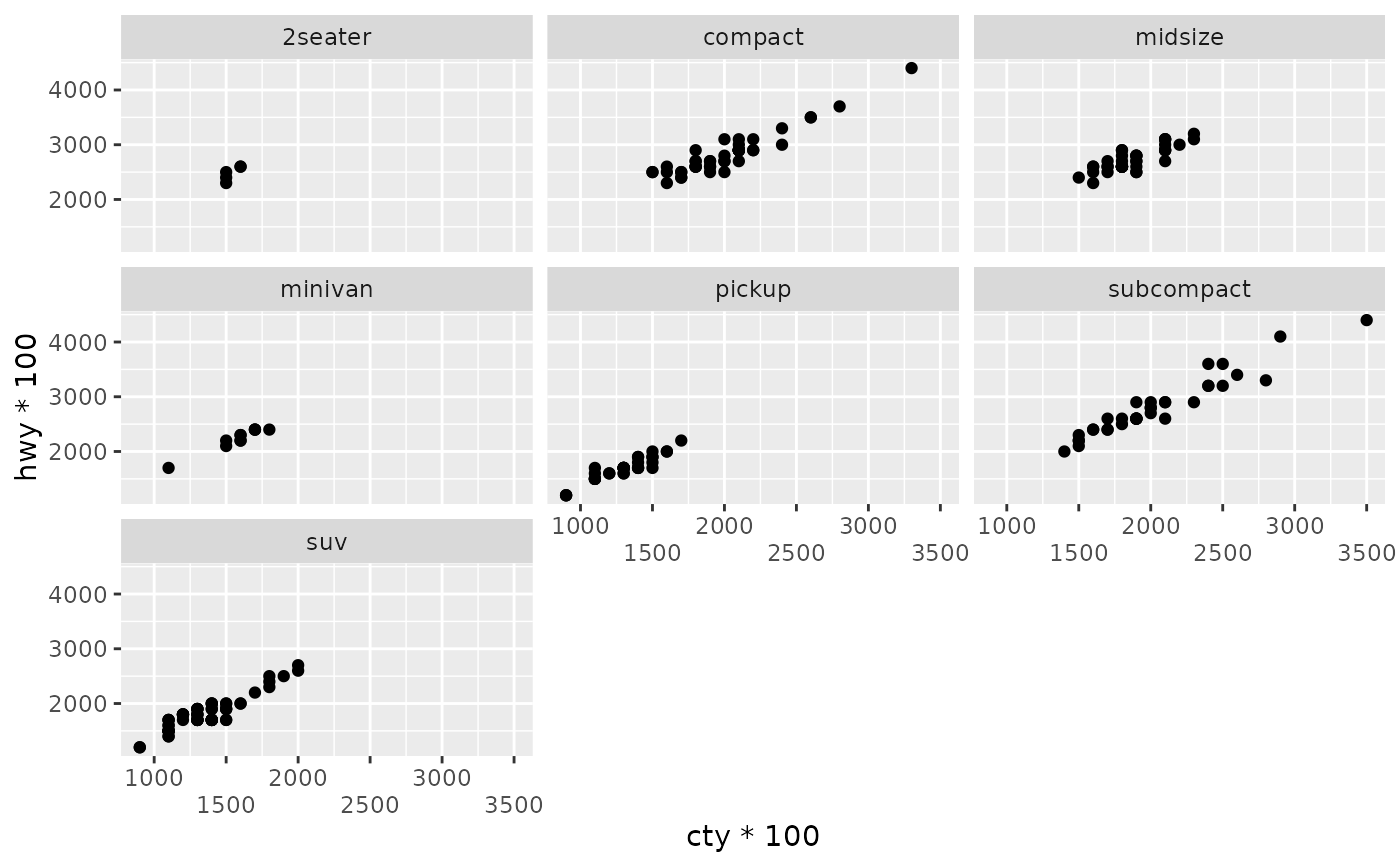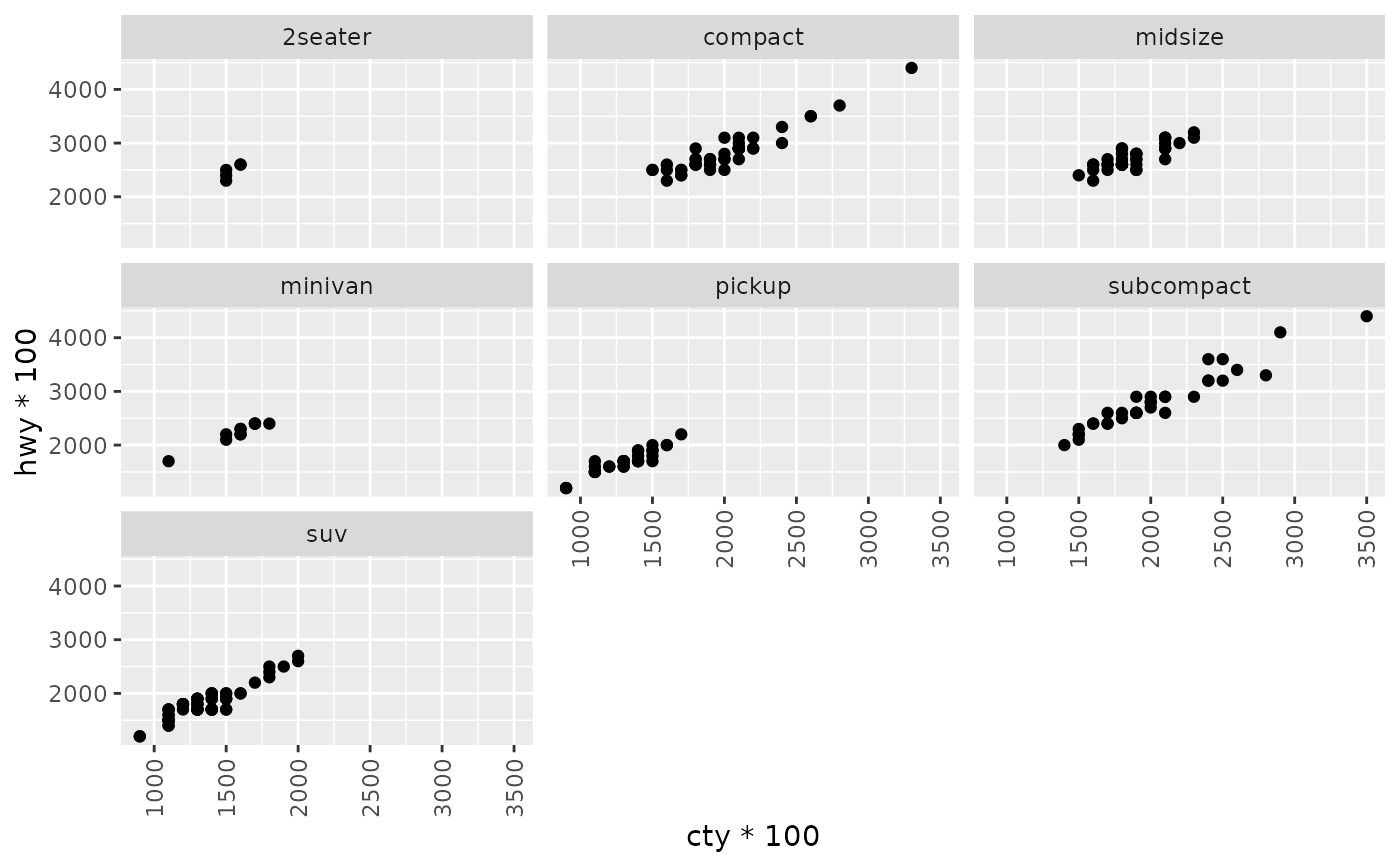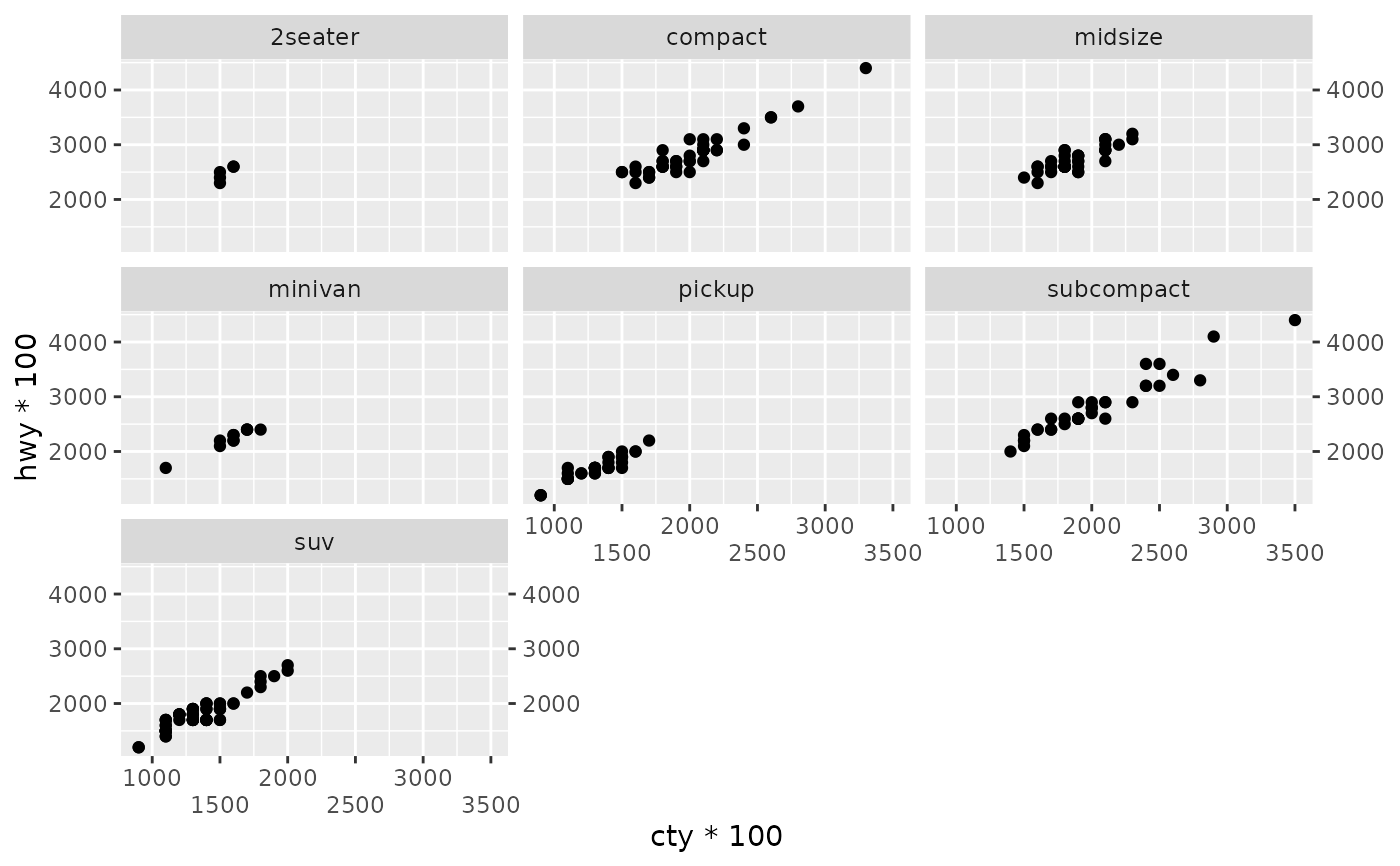Axis guides are the visual representation of position scales like those created with scale_(x|y)_continuous() and scale_(x|y)_discrete().

## Usage

guide_axis(
title = waiver(),
check.overlap = FALSE,
angle = NULL,
n.dodge = 1,
cap = "none",
order = 0,
position = waiver()
)

## Arguments

title

A character string or expression indicating a title of guide. If NULL, the title is not shown. By default (waiver()), the name of the scale object or the name specified in labs() is used for the title.

check.overlap

silently remove overlapping labels, (recursively) prioritizing the first, last, and middle labels.

angle

Compared to setting the angle in theme() / element_text(), this also uses some heuristics to automatically pick the hjust and vjust that you probably want.

n.dodge

The number of rows (for vertical axes) or columns (for horizontal axes) that should be used to render the labels. This is useful for displaying labels that would otherwise overlap.

cap

A character to cut the axis line back to the last breaks. Can be "none" (default) to draw the axis line along the whole panel, or "upper" and "lower" to draw the axis to the upper or lower break, or "both" to only draw the line in between the most extreme breaks. TRUE and FALSE are shorthand for "both" and "none" respectively.

order

A positive integer of length 1 that specifies the order of this guide among multiple guides. This controls in which order guides are merged if there are multiple guides for the same position. If 0 (default), the order is determined by a secret algorithm.

position

Where this guide should be drawn: one of top, bottom, left, or right.

## Examples

# plot with overlapping text
p <- ggplot(mpg, aes(cty * 100, hwy * 100)) +
geom_point() +
facet_wrap(vars(class))

# axis guides can be customized in the scale_* functions or
# using guides()
p + scale_x_continuous(guide = guide_axis(n.dodge = 2))p + guides(x = guide_axis(angle = 90))# can also be used to add a duplicate guide
p + guides(x = guide_axis(n.dodge = 2), y.sec = guide_axis())